# Bar Bending Schedule for footings | Steel Estimation for footings

Bar Bending Schedule for Footings, Steel Quantity for Footings, Estimation of Reinforcement in Footing

0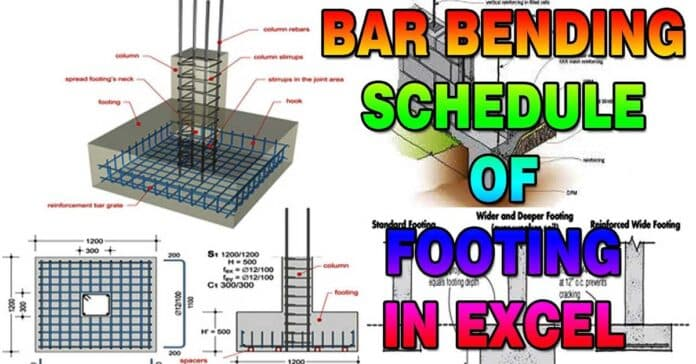# Bar Bending Schedule for Footings | Steel Quantity of Footing | Estimation of Reinforcement in Footing | BBS of Rectangular Footing

In this Article today we will talk about the Bar Bending Schedule for Footings | Steel Quantity for Footings | Estimation of Reinforcement in Footing | BBS of Rectangular Footing | Steel Estimation for Footings | Footing Estimate Calculation

## Bar Bending Schedule for footings:

Bar Bending Schedule plays a vital role in the construction of High rise buildings.  It is very important to learn Bar Bending Schedule for finding out the quantities of Steel reinforcement required for every component of the building.

For Suppose, consider the case of high rise buildings, It requires tons of steel to complete 10+ floor building. It’s impossible to order all the steel required for whole construction at a time it creates a problem of space and also steel is prone to corrosion by the contact of water (rain). To avoid this, high rise building orders reinforcement (steel) as per requirement. Firstly they find the Estimation of Steel reinforcement in footings (steel quantities)  [Bar Bending Schedule for footings],  required for the construction of footings. After the completion of footings they go for next order and so on.

If you are new to Bar Bending Schedule, Refer the Basics of Bar Bending schedule

If you wanted to know different types of footings check here Different types of footings

## Quantity of Reinforcement (Steel) required for footings / Bar Bending Schedule for footings:

The procedure to finding out the quantities of steel required for the footings, We are considering the below footing plan. Steel Quantity for Footings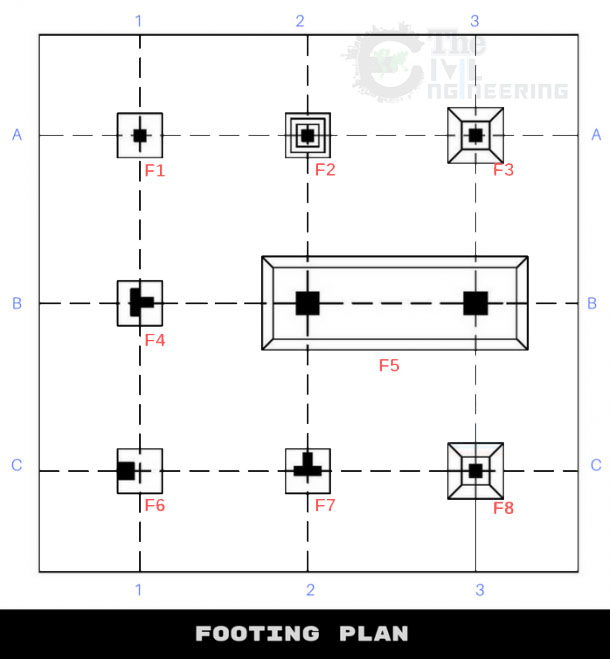Observations from the above fig:

• F1, F4, F7 is Plain footing (1.0 × 1.0 × 0.8)
• F2 is Stepped Footing (0.9 × 0.9 × 1.35)
• F3,  F8  is Isolated footing (0.9 × 0.9 × 0.5)
• F5 is Combined Isolated footing (4.2 × 1.7 × 0.9)
• F6 is Shoe footing (0.6 × 0.6 × 0.4)
True dimensions and shapes of the footings are decided and designed by the structural engineer based on soil history, type of construction, the total expected load of the structure. All the dimensions of the above columns considered only for explanation purpose. Steel Quantity for Footings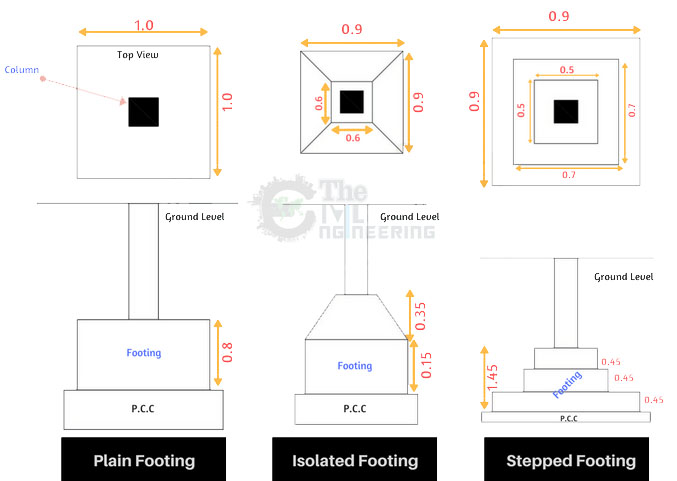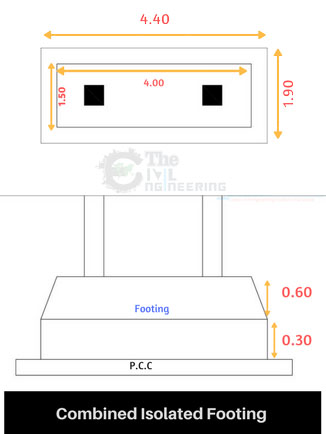We use different types of mesh (reinforcement) in footings as per design. Steel Quantity for Footings

## Steps involved in calculating the bar bending schedule of a footings:

Remember, Steel required for construction is ordered in Kgs or Number of Bars. The standard size of each bar is 12m. The final output of BBS calculation is in Kgs or in Number of “12m” Bars.

To make it easier calculation is divided into two parts, X bar Calculations and Y bar calculations. Estimation of Reinforcement in Footing

X Bars are Horizontal bars in X direction and Y Bars are vertical one projected in Y Direction. Estimation of Reinforcement in Footing
1. Deduct the concrete cover to find the dimensions of bars.
2. Find the Length of single X Bars & Y Bars
3. Find the total length of X bars. & Y bars
4. Calculate the weight of steel required per 1m
5. Calculate the total number of 12m bars required
6. Find the total weight of steel required.

### Plain footing Bar Bending schedule | F1, F4, F7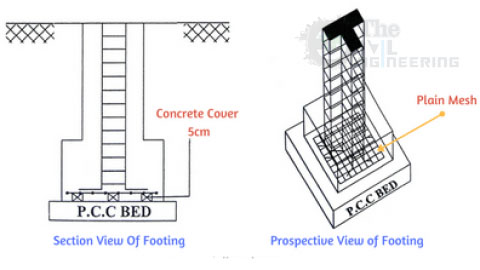For the calculation of the total quantity of steel required for the Plain footing, we are adopting these dimensions for bars.

• Dimensions of Footing  are 1.0 × 1.0 × 0.9 (Length × Breadth × Depth)
• Plain mesh is adopted for F1, F4, F7 footings
• Dia of X Bars is 16mm (Dia 16mm @ 100mm  C/C)
• Dia of Y Bars is 12mm (Dia 12mm @ 100mm C/C)
• which means Center to center spacing between X bars & Y bars is 100mm
Remember, Proper Concrete cover should be adopted for the reinforcement  in Footings to resist it from corrosion. Estimation of Reinforcement in Footing

Concrete Cover deduction:

As per condition, concrete cover of  0.1m is deducted from all sides of  mesh. True dimensions post deducting is 0.8×0.8 (length and breadth) Refer below image for more details: Estimation of Reinforcement in Footing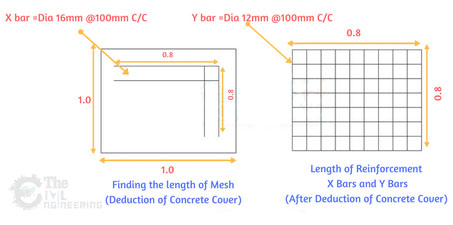Length of   Each X bar = 0.8m Length of   Each Y bar = 0.8m No. of X bars [(Y Bar Length)/Spacing]+1   = [0.8/0.1]+1 = 9bars No. of Y bars [(X Bar Length)/Spacing]+1   = [0.8/0.1]+1 = 9bars Total Length   of X bars = Length of each X bar ×   No. of X Bars = 0.8 × 9 =7.2m Total Length   of Y bars = Length of each Y bar ×   No. of Y Bars = 0.8 × 9 =7.2m Total No. of ’12m’   X bars = 7.2/12   = 0.6bars Total No. of ’12m’   Y bars = 7.2/12   = 0.6bars Weight of steel   required for 1m of 16mm bar = D2/162   = 162/162 = 1.58kg/m Total weight of   steel required for X bars = 1.58 × 7.2   = 11.37Kgs Weight of steel   required for 1m of 12mm bar = D2/162   = 122/162 = 0.88kg/m Total weight of   steel required for Y bars = 0.88 × 7.2   = 6.33Kgs

Total Weight of Plain Mesh
= Weight of steel required for X bars + Weight of steel required for Y bars
= 11.37Kgs+6.33Kgs =17.70Kgs

### Isolated footings Bar Bending Schedule | F3, F8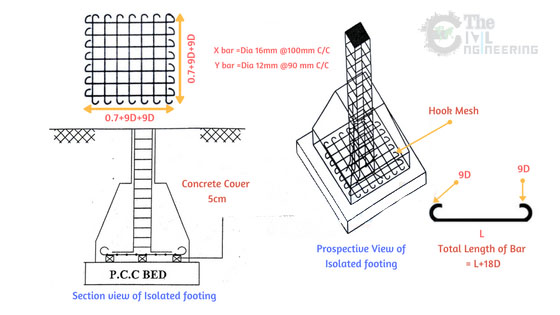For the calculation of the total quantity of steel required for the Isolated footing, we are adopting below dimensions for bars.

• Hook mesh is adopted for F3, F8 footings
• Dimensions of Footing  are 0.9×0.9×0.5 (Length × Breadth × Depth)
• Dia of X Bars is 16mm (Dia 16mm @ 100mm  C/C)
• Dia of Y Bars is 12mm (Dia 12mm @ 90mm C/C)
• which means Center to center spacing between X bars is 100mm & Y bars is 90mm
Remember Proper Concrete cover should be adopted for the reinforcement  in Footings to resist it from corrosion. BBS of Rectangular Footing

Concrete Cover deduction:

As per condition, concrete cover of  0.1m is deducted from all sides of  mesh. In hook mesh, hook is provided at the end of each bar. Each bar has two ends and therefore, hook length is included in the calculation of length of bar

Hook Length = 9d (d is the dia of bar)

Total Hooks for each bar = 2 Steel Quantity for Footings

True dimensions post deducting concrete cover is length = (0.7m+2x9d) & breadth = (0.7m+2x9d)

Refer below image for more details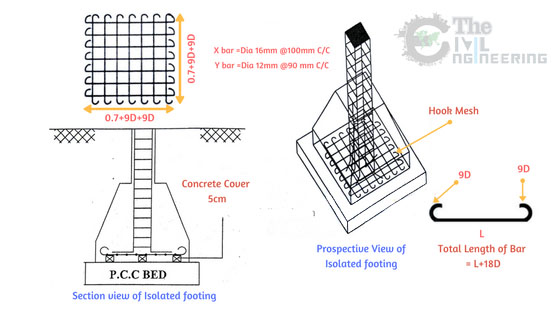Length of   Each X bar = 0.7+2×9d   d = 16mm = 0.016m = 0.7+2×9×0.016 = 0.988m Length of   Each Y bar = 0.7+2×9d   d = 12mm = 0.012m = 0.7+2×9×0.012 = 0.916m No. of X bars [(Y Bar Length)/Spacing]+1   = [0.7/0.1]+1 = 8bars (Don’t include hook length in calculating no. of bars) No. of Y bars [(X Bar Length)/Spacing]+1   = [0.7/0.09]+1 = 9bars Total Length   of X bars = Length of each X bar ×   No. of X Bars = 0.988 × 8 =7.9m Total Length   of Y bars = Length of each Y bar ×   No. of Y Bars = 0.916 × 9 =8.24m Total No. of ’12m’   X bars = 7.9/12   = 0.65bars Total No. of ’12m’   Y bars = 8.24/12   = 0.68bars Weight of steel   required for 1m of 16mm bar = D2/162   = 162/162 = 1.58kg/m Total weight of   steel required for X bars = 1.58 × 7.9   = 12.48Kgs Weight of steel   required for 1m of 12mm bar = D2/162   = 122/162 = 0.88kg/m Total weight of   steel required for Y bars = 0.88 × 8.24   = 7.25Kgs

Total Weight of Hook Mesh
= Weight of steel required for X bars + Weight of steel required for Y bars
= 12.48Kgs+7.25Kgs =19.73Kgs

### Stepped footings Bar Bending Schedule | F2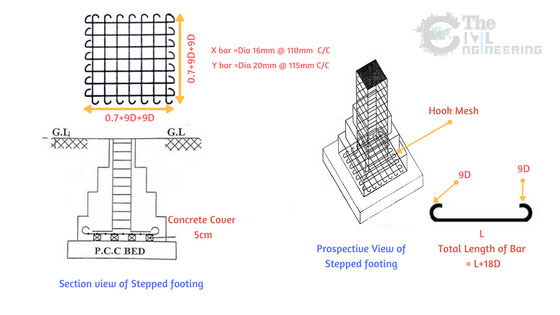For the calculation of the total quantity of steel required for the Plain footing, we are adopting these dimensions for bars.
• Hook mesh is adopted for F2 footings
• Dimensions of Footing  are 0.9 × 0.9 × 1.35 (Length × Breadth × Depth)
• Dia of X Bars is 16mm (Dia [email protected]  C/C)
• Dia of Y Bars is 20mm (Dia [email protected] C/C)
• which means Center to center spacing between X bars is 110mm & Y bars is 115mm
Remember Proper Concrete cover should be adopted for the reinforcement  in Footings to resist it from corrosion. Estimation of Reinforcement in Footing

Concrete Cover deduction:

As per condition, concrete cover of  0.1m is deducted from all sides of  mesh. In hook mesh, hook is provided at the end of each bar. Each bar has two ends and therefore, hook length is included in the calculation of length of bar

Hook Length = 9d (d is the dia of bar)

Total Hooks for each bar = 2 BBS of Rectangular Footing

True dimensions post deducting concrete cover is length = (0.7m+2×9d) & breadth = (0.7m+2×9d) BBS of Rectangular Footing

Refer below image for more details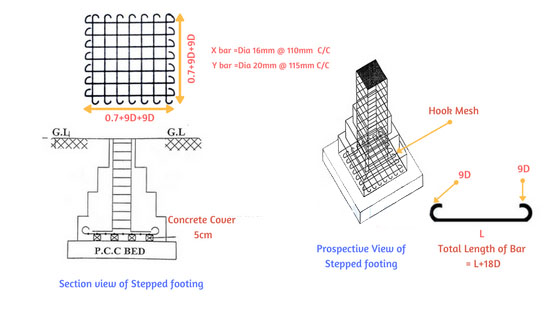Length of   Each X bar = 0.7+2×9d   d = 16mm = 0.016m = 0.7+2×9×0.016 = 0.988m Length of   Each Y bar = 0.7+2×9d   d = 12mm = 0.012m = 0.7+2×9×0.020 = 1.06m No. of X bars [(Y Bar Length)/Spacing]+1   = [0.7/0.11]+1 =~7bars (Don’t include hook length in calculating no. of bars) No. of Y bars [(X Bar Length)/Spacing]+1   = [0.7/0.115]+1 = ~6bars Total Length   of X bars = Length of each X bar ×   No. of X Bars = 0.988 × 7 =7.9m Total Length   of Y bars = Length of each Y bar ×   No. of Y Bars = 1.06 × 6 =6.36m Total No. of ’12m’   X bars = 7.9/12   = 0.65bars Total No. of ’12m’   Y bars = 6.36/12   = 0.53bars Weight of steel   required for 1m of 16mm bar = D2/162   = 162/162 = 1.58kg/m Total weight of   steel required for X bars = 1.58 × 7.9   = 12.48Kgs Weight of steel   required for 1m of 12mm bar = D2/162   = 202/162 = 2.46kg/m Total weight of   steel required for Y bars = 2.46 × 6.36   = 15.64Kgs

Total Weight of Hook Mesh
= Weight of steel required for X bars + Weight of steel required for Y bars
= 12.48Kgs+15.64Kgs =28.12Kgs

### Eccentric/Shoe footings Bar Bending Schedule | F6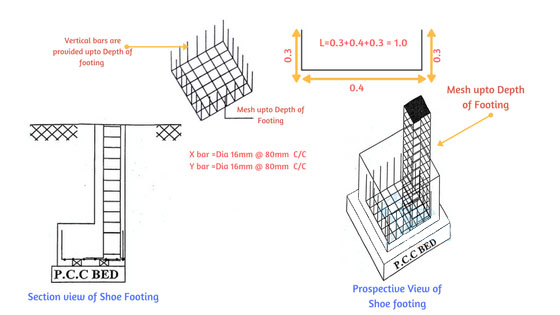For the calculation of the total quantity of steel required for the Plain footing, we are adopting these dimensions for bars. BBS of Rectangular Footing

• Mesh extended till Depth of Footing is adopted for F6 footings
• Dimensions of Footing  are 0.6 × 0.6 × 0.4 (Length × Breadth × Depth)
• Dia of X Bars is 16mm (Dia [email protected]  C/C)
• Dia of Y Bars is 16mm (Dia [email protected] C/C)
• which means Center to center spacing between X bars & Y bars is 80mm
Remember Proper Concrete cover should be adopted for the reinforcement  in Footings to resist it from corrosion. BBS of Rectangular Footing

Concrete Cover deduction:

As per condition, concrete cover of  0.1m is deducted from all sides of  mesh. In Mesh extended till Depth of Footing, extra bar is bent towards the depth.
 Length of   Each X bar = 0.4+0.3+0.3   = 1.0m Length of   Each Y bar = 0.4+0.3+0.3   = 1.0m No. of X bars [(Y Bar Length)/Spacing]+1   = [0.4/0.08]+1 =~6bars (Don’t include extra bar length in calculating no. of bars) No. of Y bars [(X Bar Length)/Spacing]+1   = [0.4/0.08]+1 = ~6bars Total Length   of X bars = Length of each X bar ×   No. of X Bars = 1.0 × 6 =6m Total Length   of Y bars = Length of each Y bar ×   No. of Y Bars = 1.0 × 6 =6m Total No. of ’12m’   X bars = 6/12   = 0.5bars Total No. of ’12m’   Y bars = 6./12   = 0.5bars Weight of steel   required for 1m of 16mm bar = D2/162   = 162/162 = 1.58kg/m Total weight of   steel required for X bars = 1.58 × 6   = 9.48Kgs Weight of steel   required for 1m of 12mm bar = D2/162   = 122/162 = 1.58kg/m Total weight of   steel required for Y bars = 1.58 × 6   = 9.48Kgs

Total Weight of Mesh extended till Depth of Footing
= Weight of steel required for X bars + Weight of steel required for Y bars =9.48Kgs+9.48Kgs =18.96Kgs

### Combined Footing Bar Bending Schedule | F5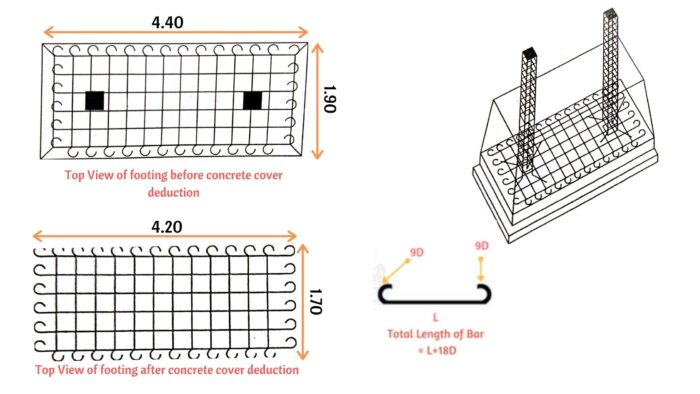For the calculation of the total quantity of steel required for the combined footing, we are adopting these dimensions for bars. Steel Estimation for Footings

• Hook Mesh is usually adopted for F5 footing.
• Dimensions of Footing  are 4.2 × 1.7 × 0.9 (Length × Breadth × Depth)
• Dia of X Bars is 12mm (Dia [email protected]  C/C)
• Dia of Y Bars is 12mm (Dia [email protected] C/C)
• which means Center to center spacing between X bars & Y bars is 100mm
Remember Proper Concrete cover should be adopted for the reinforcement  in Footings to resist it from corrosion. Steel Estimation for Footings

Concrete Cover deduction:

As per condition, concrete cover of  0.1m is deducted from all sides of  mesh. In Mesh extended till Depth of Footing, extra bar is bent towards the depth.
 Length of   Each X bar = 4.2+2×9d   d = 12mm = 0.012m = 4+2×9×0.012 = 4.41m Length of   Each Y bar = 1.7+2×9d   d = 12mm = 0.012m = 1.7+2×9×0.012 = 1.91m No. of X bars [(Y Bar Length)/Spacing]+1   = [1.7/0.1]+1 =18bars (Don’t include hook length in calculating no. of bars) No. of Y bars [(X Bar Length)/Spacing]+1   = [4.2/0.1]+1 = 43bars Total Length   of X bars = Length of each X bar ×   No. of X Bars = 4.41 × 18 =79.38m Total Length   of Y bars = Length of each Y bar ×   No. of Y Bars = 1.91 × 43 =82.13m Total No. of ’12m’   X bars = 79.38/12   = 6.6bars Total No. of ’12m’   Y bars = 82.13/12   = 6.84bars Weight of steel   required for 1m of 16mm bar =D2/162   =122/162 =0.88kg/m Total weight of   steel required for X bars = 0.88 × 79.38   = 69.85Kgs Weight of steel   required for 1m of 12mm bar =D2/162   =122/162 =0.88kg/m Total weight of   steel required for Y bars = 0.88 ×82.13   = 72.27Kgs

Total Weight of Hook Mesh
= Weight of steel required for X bars + Weight of steel required for Y bars
= 69.85Kgs + 72.27Kgs =142.12Kgs

### Abstract of above calculation: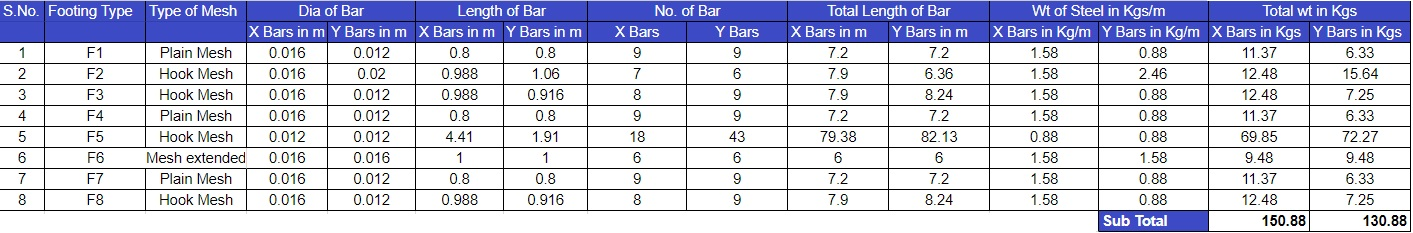Total Weight of steel required for above plan = Total weight of steel required for X bars +Total weight of steel required for Y bars = 150.88 Kgs + 130.88 Kgs = 281.76 Kgs = 0.28 Ton

## Conclusion:

Full article on Bar Bending Schedule for Footings | Steel Quantity for Footings | Estimation of Reinforcement in Footing | BBS of Rectangular Footing | Steel Estimation for Footings | Footing Estimate Calculation Thank you for the full reading of this article in “The Civil Engineering” platform in English. If you find this post helpful, then help others by sharing it on social media. If you have any question regarding article please tell me in comments.